Arens regularity

A normed algebrais said to be Arens regular if the pair of intrinsically defined Arens products (introduced by R. Arens in [a1] and [a2]; cf. Arens multiplication) on the double dual spaceare identical. Since both Arens products extend the product on(relative to the natural embedding mapping), a Banach algebra on a reflexive Banach space (cf. also Reflexive space) is Arens regular. S. Sherman has shown [a10] that the double dual of a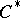-algebrahas a natural interpretation as the von Neumann algebra generated by the universal-representation of. Hence-algebras are always Arens regular.

It is easy to show that if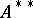is commutative under either Arens product, thenis Arens regular. The following fundamental result is due to J. Hennefeld [a5], based on work of J.S. Pym [a8] making use of Grothendieck's criterion for weak compactness.

The following conditions are equivalent for a Banach algebra:

a)is Arens regular;

b) for each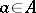the adjointof the left regular representation is weakly compact;

c) for eachthe adjointof the right regular representation is weakly compact;

d) for any bounded sequences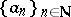and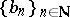inand any, the iterated limits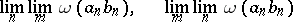are equal when they both exist.

This theorem easily implies that subalgebras and quotient algebras (with respect to closed ideals) of Arens-regular algebras are Arens regular, as first noted in [a3].

Arens regularity is rare among general Banach algebras. N.J. Young [a11] has shown that for a locally compact group (cf. also Compact group; Locally compact skew-field),(or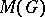) is Arens regular if and only ifis finite. P. Civin and B. Yood had proved this for Abelian groups in [a3]. In [a12] it is shown that the measure algebra (cf. Algebra of measures)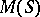of a locally compact semi-groupin which multiplication is at least singly continuous is Arens regular if and only if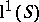is. These are, in turn, equivalent to either:

there do not exist sequences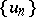andinsuch that the setsand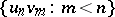are disjoint;

the semi-group operation can be extended to the Stone–Čech compactification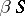ofas a discrete space.

In [a13], Young has proved that the algebraof approximable operators (i.e., those uniformly approximable by finite-rank operators) on a Banach spaceis regular if and only ifis reflexive (cf. Reflexive space). Hence, if the Banach algebraof all bounded linear operators on a Banach spaceis Arens regular, thenmust be reflexive. He also shows that there are reflexive Banach spaceswith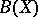not Arens regular.

Á. Rodriguez-Palacios [a9] has shown that any (even non-associative) continuous multiplication on a Banach spaceis Arens regular if and only if every bounded linear mapping fromintois weakly compact (cf. Weak topology).-algebras satisfy this criterion.

A weaker version of Arens regularity was introduced by M. Grosser [a4]. An approximately unital Banach algebrais said to be semi-regular if it satisfiesfor all mixed identities. (An elementis called a mixed identity if it is simultaneously a right identity for the first Arens product and a left identity for the second Arens product, see Arens multiplication.) He shows that an Arens-regular algebra is semi-regular and that any commutative approximately unital Banach algebra is semi-regular.

The most comprehensive recent (1996) exposition is [a7], which contains numerous further references.